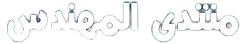# موضوع فوق الممتاز عن القدرة و المقاومة وكيفية حساب Propeller Pitch للسفن

[CENTER] موضوع فوق الممتاز عن القدرة و المقاومة وكيفية حساب Propeller Pitch للسفن

ملخص الكتاب :

1. Be able to define effective horsepower (EHP) physically and mathematically.
2. Be able to state the relative between velocity with total resistance and velocity with effective horsepower.
3. Be able to write an equation for total hull resistance as a sum of viscous resistance, wave making resistance and correlation resistance. Be able to physically explain each of these resistive terms.
4. Be able to draw and explain the flow of water around a moving ship showing laminar flow region, turbulent flow region, and separated flow region.
5. Be able to draw the transverse and longitudinal wave patterns when a displacement ship moves through the water.
6. Be able to define the Reynolds number with a mathematical formula. Be able to explain each parameter in the Reynolds equation with units.
7. Be qualitatively familiar with the following minor sources of ship resistance: a. Steering Resistance b. Air and Wind Resistance c. Added Resistance due to Waves d. Increased Resistance in Shallow Water
8. Read and interpret a ship resistance curve including humps and hollows.
9. Be able to state the importance of naval architecture modeling of the resistance on the ship’s hull.
10. Be able to define geometric and dynamic similarity.
11. Be able to write the relationships for geometric scale factor in terms of length ratios, speed ratios, wetted surface area ratios or volume ratios.
12. Be able to describe the law of comparison (Froude’s law of corresponding speeds) physically and mathematically and state its importance in model testing

وهذا هو الرابط :

وهذا هو الرابط :

رغم انى مش بفهم فى هندسة السفن
لكن بارك الله لك